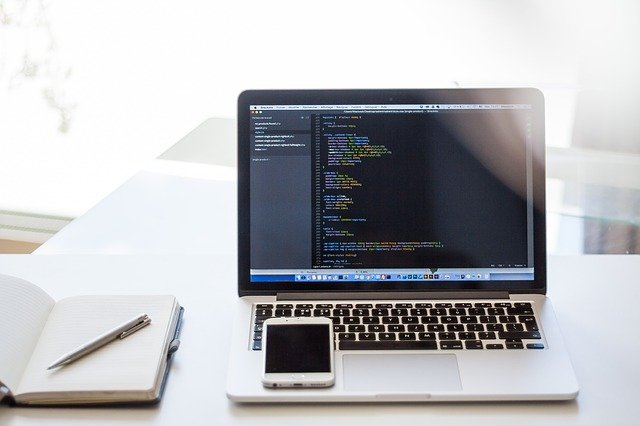# Corporate Finance Management

Solution Coupon rate = 7% = 3.5%

face value of the bond = \$1000

Yield on the bond = 13%, i.e. 6.5%

Semi annually N = mo. of years to maturity = 15 years. = 30

But, 6, 7 and 8 year company will skip coupon payment

Value of Interest Payments =

Value of the bond =

= 3.5% * 1000 * -368.16 + 22535.21

Corporate bond’s value = 9649.61

In the presented scenario, there is supernormal growth. But at the same time, dividend growth rate requires to change each year. That is why, three year of share price can be calculate sicne the divided growth is constant. In this way, the stock price will be the 4 years dividend. Hence, constant dividend growth rate will be less from the required return. Therefore, price of stock in three years will be:

P3 = \$4.00 (1.20) (1.10) (1.15) / (0.15 – 0.05)    (Arnold, 2013).

= \$60.72

In this, it can be understand that price of the stock today will be PV the initial three years, plus of the stock price in year 3

Po = 4.00(1.20)/(1.15) + 4.00(1.20)(1.15)/1.152 + 4.00(1.20) (1.15) (1.10)/1.153 + 60.72/1.153

Po = 52.26 (Mac Innis, 2013)

Today price of the stick will be 52.26

1. Net Present Value

The net present value shows the present value of the investment that is calculated in the different ways. In this, main ways of calculating the NPV is expected cash flow minus the cost of acquiring the investment. The rule of NPV says that go ahead with a project, as the investment should result in a net gain in profitability and value for the company. The NPV rule also says that the investment in a project that has a net present value is high than zero determines that project is profitable (Wood, 2016). The NPV of this project is below:

First way of calculating NPV

Investment = \$6500000

Cash flow = \$15000000

NPV = 15000000 – 6500000

NPV = \$8500000

Second way of calculating NPV

 Net Present value Year Cash Inflow PV factor @10% Present value Initial cost 6500000 _ 6500000 Cash flow in 1st Year 3000000 0.909 2727000 Cash flow in 2st Year 3000000 0.826363636 2479090.91 Cash flow in 3st Year 3000000 0.751239669 2253719.01 Cash flow in 4st Year 3000000 0.682945154 2048835.46 Cash flow in 5st Year 3000000 0.620859231 1862577.69 NPV 17871223.07

From the above calculation, it can be seen that the value of NPV is good in the both ways that is why, it can be said that investment in this project will be profitable for company.

1. Change in the value of firm

It has already determined that contract will be profitable for company. If the company will take this project then it value will be increase by the value of the NPV after the completion of the contract.

1. Payback period

In the financial management, payback period defines that number of year that shows how much time will be taken in recovering the investment money in project.

Payback period = Initial Investment / cash flow per year    (Meier & Schier, 2012)

Initial investment = \$6500000

Cash flow per year = \$3000000

Payback period = 6500000 / 3000000

= 2.16 Years

Standard deviation of portfolio = 30%

Expected return of portfolio = 18%

By adding stock A = 15% * 25%* 0.2

= 0.0075

By adding stock B = 15% * 20% * 0.6

= 0.018

1. SD [A] = 0.2548;

SD [B] = 0.2659 (choose A) (Nejad & Kermani, 2012)

On the basis of calculation, it is found that the portfolio holder should add the stock of A. It is because it will provide more return as well as provide the flexibility of adding 20% money in new stock and 80% money in the existing stock.

Intel’s beta = 1.8

Boeing’s beta = 1.2

Risk free rate = 5%

Expected return of the market portfolio = 15%

Expected rate of return from Intel = 20%

Expected rate of return from Boeing = 25%

Based on CAPM = E(R) = RFR + β(RM –RFR  (Mac Innis, 2013).

RFR= Risk free rate

B = Beta of the stock

RM = Expected return of the market portfolio

A: Intel’s Expected Return

= 5 + 1.8 (15-5)

= 23%

B: Boeing’s Expected Return

= 5 + 1.2 (15-5)

= 17%

1. Beta of the portfolio

Beta of the portfolio = % of fist company (its beta) + % of the second company (its beta) (Wood, 2016).

= 0.7 (1.8) + 0.3 (1.2)

= 1.62

1. Investment Decision

From the above results, it can be recommended that Intel stock should buy and Boeing stock should sell. It is because Intel (23%) has more expected return as compared to Boeing (17%).

Apple’s beta = 1.2

Risk-free = 3%

Beginning Apple’s price = \$75

Closing Apple’s price \$80

CAPM = rf + Ba (rm – rf) (Meier & Schier, 2012)

rf = Risk free rate

Ba = Beta of the stock

rm = Expected market return

(rm – rf) is also known as market risk premium

= 0.03 + 1.2 (0.06)

= 10.20%

Hence, it can be said that Apple’s managers significantly exceeded the required return of investors, according to CAPM. It is because, CAPM result is found 10.2% increase.

References

Arnold, G. (2013). Corporate financial management. UK: Pearson Higher Ed.

Mac Innis, J. P. (2013). U.S. Patent No. 8,392,294. Washington, DC: U.S. Patent and Trademark Office.

Meier, O., & Schier, G. (2012). Fusions acquisitions-4e 2d.: Stratégie. Finance. Management. UK: Dunod.

Nejad, H. R., & Kermani, S. A. (2012). The relation between insurance development and economic growth in Iran. Finance Management47, 9079-9087.

Wood, D. A. (2016). Comparing the publication process in accounting, economics, finance, management, marketing, psychology, and the natural sciences. Accounting Horizons30(3), 341-361.